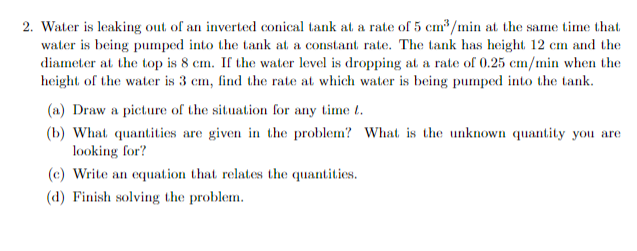# Question 2. Water is leaking out of an inverted conical tank at a rate of $$5 \mathrm{~cm}^{3} / \mathrm{min}$$ at the same time that, water is being pumped into the tank at, a constant, rate. The tank has height. $$12 \mathrm{~cm}$$ and the diameter at the top is $$8 \mathrm{~cm}$$. If the water level is dropping at a rate of $$0.25 \mathrm{~cm} / \mathrm{min}$$ when the height, of the water is $$3 \mathrm{~cm}$$, find the rate at which water is being pumped into the tank. (a) Draw a picture of the situation for any time $$t$$. (b) What quantities are given in the problem? What is the unknown quantity you are looking for? (c) Write an equation that relates the quantities. (d) Finish solving the problem.Transcribed Image Text: 2. Water is leaking out of an inverted conical tank at a rate of $$5 \mathrm{~cm}^{3} / \mathrm{min}$$ at the same time that, water is being pumped into the tank at, a constant, rate. The tank has height. $$12 \mathrm{~cm}$$ and the diameter at the top is $$8 \mathrm{~cm}$$. If the water level is dropping at a rate of $$0.25 \mathrm{~cm} / \mathrm{min}$$ when the height, of the water is $$3 \mathrm{~cm}$$, find the rate at which water is being pumped into the tank. (a) Draw a picture of the situation for any time $$t$$. (b) What quantities are given in the problem? What is the unknown quantity you are looking for? (c) Write an equation that relates the quantities. (d) Finish solving the problem.
Transcribed Image Text: 2. Water is leaking out of an inverted conical tank at a rate of $$5 \mathrm{~cm}^{3} / \mathrm{min}$$ at the same time that, water is being pumped into the tank at, a constant, rate. The tank has height. $$12 \mathrm{~cm}$$ and the diameter at the top is $$8 \mathrm{~cm}$$. If the water level is dropping at a rate of $$0.25 \mathrm{~cm} / \mathrm{min}$$ when the height, of the water is $$3 \mathrm{~cm}$$, find the rate at which water is being pumped into the tank. (a) Draw a picture of the situation for any time $$t$$. (b) What quantities are given in the problem? What is the unknown quantity you are looking for? (c) Write an equation that relates the quantities. (d) Finish solving the problem.&#12304;General guidance&#12305;The answer provided below has been developed in a clear step by step manner.Step1/2sorry that is mistaken ly posted please reconsider $$\mathrm{{663}+}$$Explanationsorry that is mistaken ly ... See the full answer